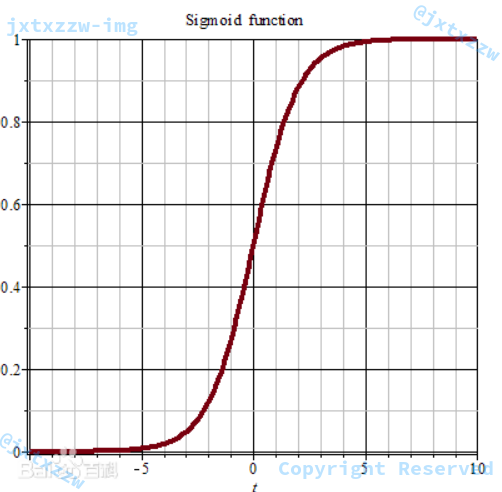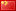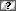## 使用逻辑回归对数据集进行多分类

3371 9 2018年11月11日

5 1 投票

## 逻辑回归用来分类

• $p$ 的取值范围是 $[0,1]$，而等式右边是 $(-\infty,+\infty)$
• 实际中很多问题，概率 $p$ 与自变量并不是直线关系

$\displaystyle P(y=1|x)=\frac{e^{\theta^{T}x}}{1+e^{\theta^{T}x}}=\frac{1}{1+e^{-\theta^{T}x}}$

$\displaystyle P(y=0|x)=\frac{1}{1+e^{\theta^{T}x}}$

## Sigmoid 函数## 损失函数

$\displaystyle J(\theta)=-m^{-1}l(\theta)=-m^{-1}\sum_{i}^{m}{[y^{(i)} \ln h_{\theta}(x^{(i)})+(1-y^{(i)}) \ln ( 1-h_{\theta}(x^{(i)}) )]}$

## 随机梯度下降

• 初始化最初解
• 循环直至收敛

$\displaystyle \theta_j = \theta_j – \alpha \frac{\partial{J}}{\partial{\theta_j}}=\theta_j-\alpha(h_{\theta}(x^{(i)})-y^{(i)})x_j^{(i)}$

## SoftMax

$\sigma_{j}={\frac {e^{z_{j}}}{\sum {k=1}^{K}e^{z{k}}}}$

SoftMax 函数实际上是有限项离散概率分布的梯度对数归一化。

$P={\frac {e^{\mathbf {x} ^{\mathsf {T}}\mathbf {w} _{j}}}{\sum _{k=1}^{K}e^{\mathbf {x} ^{\mathsf {T}}\mathbf {w} _{k}}}}$

SoftMax 针对多类分类，输出的是每一个分类的概率。

\begin{align} h_\theta&= \frac{1}{ e^{\theta_1^Tx} + e^{ \theta_2^T x^{(i)} } } \begin{bmatrix} e^{ \theta_1^T x } \ e^{ \theta_2^T x } \end{bmatrix} \end{align}

## 核心代码

public double predict(ArrayList<Double> x) {
double[] p = new double[K];
for (int i = 0; i < K; ++i) {
for (int j = 0; j < DIM; ++j) {
p[i] += x.get(j) * weight[i][j];
}
}
int idx = 0;
double tmp = p[idx];
for (int i = 0; i < K; ++i) {
if (p[i] > tmp) {
tmp = p[i];
idx = i;
}
}
return idx;
}

for (int i = 0; i < K; ++i)
for (int j = 0; j < DIM; ++j)
p[i] += x.get(j) * weight[i][j];

 for (int i = 0; i < K; ++i)
if (p[i] > tmp) {
tmp = p[i];
idx = i;
}

for (int i = 0; i < K; ++i)
partialDerivative.add(calculatePartialDerivative(i, x, y));

for (int i = 0; i < K; ++i)
for (int j = 0; j < DIM; j++)
weight[i][j] -= learningRate * partialDerivative.get(i).get(j);

for k in range(K):
w[k] -= lr * pd[k]

theta_l = self.w[l]
product = np.dot(theta_l,x)

$\displaystyle e^{\theta_j^{T} x^{(i)}}$，可以直接用 Python 的 numpy 的dot()，或者用 numpy 的矩阵转置先求出 $\theta_j.T$ 再求。

double sumOfProduct = 0;
for (int i = 0; i < DIM; ++i) {
double xx = x.get(i);
double ww = w[i];
sumOfProduct += xx * ww;
}
return Math.exp(sumOfProduct);

private double calculateProbability(ArrayList<Double> x, int k) {
double numerator = calculateExp(x, weight[k]);
double denominator = 0;
for (int i = 0; i < K; ++i) {
denominator += calculateExp(x, weight[i]);
}
return numerator / denominator;
}

denominator = sum([calculateExp(x, i) for i in range(K)])

double probability = calculateProbability(x, k);

$\textstyle 1{\cdot}$是示性函数，其取值规则为，$\textstyle 1{值为真的表达式}=1$。

double characteristic = k == y ? 1.0 : 0.0;

- x * (characteristic - probability) + weightDecay * w[k]

double delta = (characteristic - probability);
for (int i = 0; i < x.size(); i++) {
double xx = x.get(i);
xx *= delta;
}

for (int i = 0; i < K; ++i)
partialDerivative.add(calculatePartialDerivative(i, x, y));
partialDerivative = [calculatePartialDerivative(k, x, y) for k in range(K)]

for (int i = 0; i < K; ++i)
for (int j = 0; j < DIM; j++)
weight[i][j] -= learningRate * partialDerivative.get(i).get(j);

public SoftMaxRegression(double learningRate, double weightDecay, int k, int dIM, int iTERATION_TIMES) {
this.learningRate = learningRate;
this.weightDecay = weightDecay;
K = k;
DIM = dIM;
ITERATION_TIMES = iTERATION_TIMES;
weight = new double[K][DIM];
}

public double[][] getWeight() {
return weight.clone();
}

for (int i = 0; i < len - 1; i++) {
}
double label = Double.valueOf(ss[len - 1]);
labels.add(label);

for (int i=0;i<len - 1;i++) {
}
features.add(feature);

DataSet train = new DataSet(new File("train.txt"), true);
DataSet test = new DataSet(new File("test.txt"), false);
final int K = new HashSet<Double>(labels).size();
final int DIM = features.get(0).size();
final double learningRate = 0.01;
final double weightDecay = 0.01;
final int ITERATION_TIMES = 100000;

SoftMaxRegression smr = new SoftMaxRegression(learningRate, weightDecay, K, DIM, ITERATION_TIMES);
smr.train(features, labels);
double[][] w = smr.getWeight();
System.out.println(Arrays.deepToString(w));

features = test.loadFeatures();
for (int i = 0; i< features.size();++i) {
int result = (int) smr.predict(features.get(i));
String label = "";
switch (result) {
case 0:
label = "Iris-setosa";
break;
case 1:
label = "Iris-versicolor";
break;
case 2:
label = "Iris-virginica";
break;
}
System.out.println(label);
}

## 运行结果

int number = features.size();
for (int t = 0 ; t < ITERATION_TIMES;t++) {
for (int index = 0; index < number;index++) {
ArrayList<Double> x = new ArrayList<Double>();
double y = labels.get(index);
// DO SOMETHING ...
}
}

int number = features.size();
for (int t = 0 ; t < ITERATION_TIMES;t++) {
int index = (int) (Math.random() * number);
ArrayList<Double> x = new ArrayList<Double>();
double y = labels.get(index);
// DO SOMETHING ...
}

• double learningRate
• double weightDecay
• int iTERATION_TIMES
5 1 投票

9条留言（可选）如果您也有个人网站，不妨分享一下

9 评论Zzz (@guest_3992)Edge 110.0.1587.63 Windows 10

2023年3月6日 21:29Zzz (@guest_3994)Edge 110.0.1587.63 Windows 10

2023年3月7日 20:31

@凝神长老就是核心代码那块﻿ 😂 ﻿Zzz (@guest_3995)Edge 110.0.1587.63 Windows 10

2023年3月7日 20:35

@凝神长老想要参考一下整个代码的实现﻿ 😂 ﻿Zzz (@guest_3998)Edge 110.0.1587.63 Windows 10

2023年3月8日 14:25

@凝神长老好的，谢谢，我试着实现一下Zzz (@guest_4002)Edge 110.0.1587.69 Windows 10

2023年3月14日 10:14

@凝神长老请问有数据读入的源代码吗Zzz (@guest_4003)Edge 110.0.1587.69 Windows 10

2023年3月14日 10:18

@凝神长老请问有读取数据集的源代码吗

Warning: error_log(/home/wwwroot/www.jxtxzzw.com/wp-content/plugins/spider-analyser/#log/log-2919.txt): failed to open stream: No such file or directory in /home/wwwroot/www.jxtxzzw.com/wp-content/plugins/spider-analyser/spider.class.php on line 2900